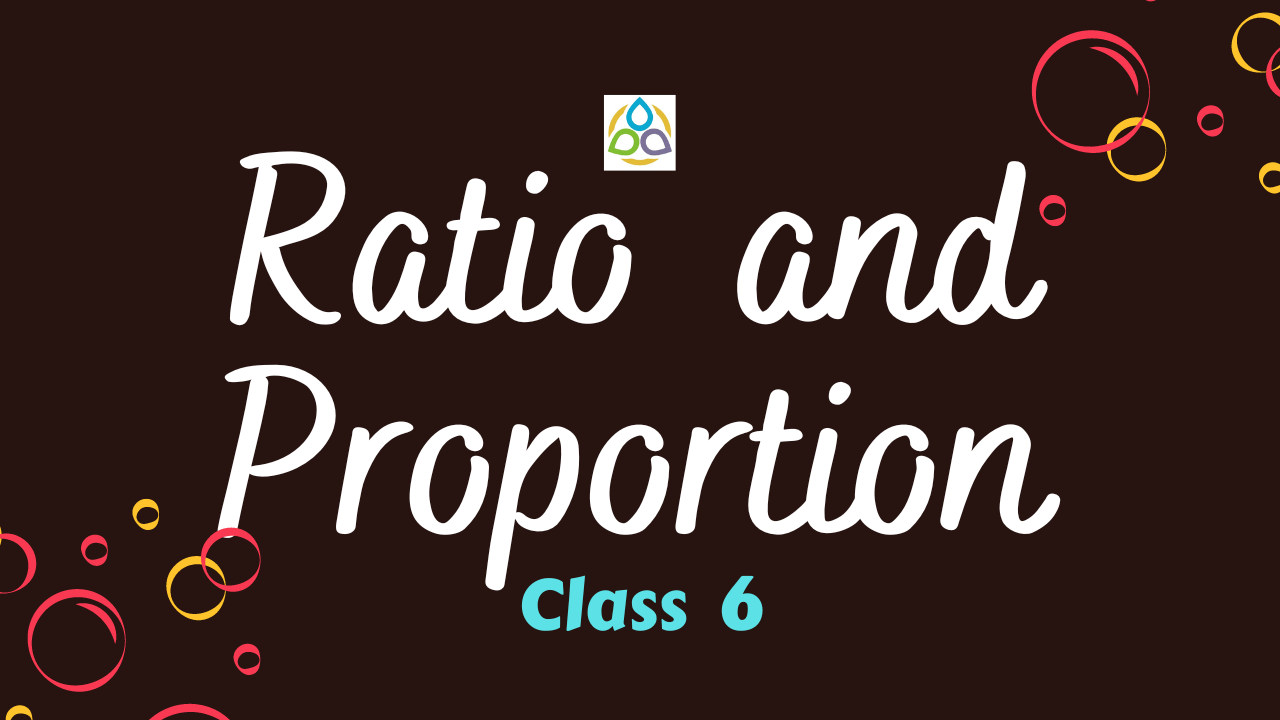# Ratio and Proportion

Multiple Choice Question

66. The comparison of two numbers or quantities by division is known as

• (A) Ratio
• (B) Proportion
• (C) Unitary method
• (D) Variation

65. Two ratios are equivalent, if the fractions corresponding to them are

• (A) Different
• (B) Equivalent
• (C) Larger
• (D) Smaller

64. Four quantities are said to be in proportion, if the ratio of the first two and the last two quantities are

• (A) Different
• (B) Same
• (C) Larger
• (D) Smaller

63. The method in which first we find the value of one unit and then the value of the required number of units is known as

• (A) Ratio
• (B) Proportion
• (C) Unitary method
• (D) Variation

62. The ratio of ₹8 to 80 paise is

• (A) 1 : 10
• (B) 10 : 1
• (C) 1: 1
• (D) 100 : 1

61. The length and breadth of a steel tape are 10 m and 2.4 cm, respectively. The ratio of the length to the breadth is

• (A) 5 : 1.2
• (B) 25 : 6
• (C) 625 : 6
• (D) 1250 : 3

60. What is the missing number in the proportion * : 8 :: 12 : 32?

• (A) 5
• (B) 3
• (C) 6
• (D) 7

59. Find the missing number in * : 18 = 28 : 84.

• (A) 5
• (B) 3
• (C) 6
• (D) 7

58. Find the missing number in 25 persons : 130 persons = 15 kg : ?

• (A) 78 persons
• (B) 78 kg
• (C) 75 kg
• (D) 75 persons

57. The ratio of 8 books to 20 books is

• (A) 2 : 5
• (B) 5 : 2
• (C) 4 : 5
• (D) 5 : 4

56. What is the missing number 3 : 33 = 33 : *?

• (A) 333
• (B) 363
• (C) 393
• (D) 303

55. What is the missing number 0.2 : 5 = * : 20?

• (A) 0.5
• (B) 0.8
• (C) 1
• (D) 1.2

54. What is the missing number 27 cm2 : 51 cm2 = 0.009 cm : *?

• (A) 0.017 cm
• (B) 0.0017 cm
• (C) 1.7 cm
• (D) 0.17 cm

53. What is the missing number * : 7.5 kg = ₹23 : ₹34.5?

• (A) 5 kg
• (B) 2.5 kg
• (C) 1.5 kg
• (D) 6.5 kg

52. What is the missing number 20 g : 100 g = * : 0.085 metre?

• (A) 0.17 metre
• (B) 0.0017 metre
• (C) 0.017 metre
• (D) 1.7 metre

51. The ratio of 1 hour to one day is

• (A) 24 : 1
• (B) 1 : 24
• (C) 1 : 60
• (D) 60 : 1

50. The ratio of 1 hour to 1 second is

• (A) 60 : 1
• (B) 1 : 60
• (C) 3600 : 1
• (D) 1 : 3600

49. Length of a pencil is 18 cm and its diameter is 8 mm. What is the ratio of the diameter of the pencil to that of its length?

• (A) 45 : 2
• (B) 2 : 45
• (C) 36 : 5
• (D) 5 : 32